Courses

# Chapter 5 Digital - to - Analog And Analog - to - Digital converters - Notes, Basic Electronics, Ele Electrical Engineering (EE) Notes | EduRev

## Electrical Engineering (EE) : Chapter 5 Digital - to - Analog And Analog - to - Digital converters - Notes, Basic Electronics, Ele Electrical Engineering (EE) Notes | EduRev

The document Chapter 5 Digital - to - Analog And Analog - to - Digital converters - Notes, Basic Electronics, Ele Electrical Engineering (EE) Notes | EduRev is a part of the Electrical Engineering (EE) Course Electrical Engineering SSC JE (Technical).
All you need of Electrical Engineering (EE) at this link: Electrical Engineering (EE)

Digital - to - Analog And Analog - to - Digital converters

A/D AND D/A CONVERTERS
Digital - to - Analog converters The input to a D/A converter is an N-bit binary signal. The analog output voltage Vo of an N-bit straight binary D/A converter is related to the digital input by the equation.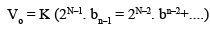where, K = Proportionality factor.
bn = 1 if the nth bit of the digital input is 1. = 0 if the nth of the digital input is 0.
Two Types of Commonly Used D/A Converters.

1. Weighted-resistor D/A converter and
2. R-2 R ladder D/A converter.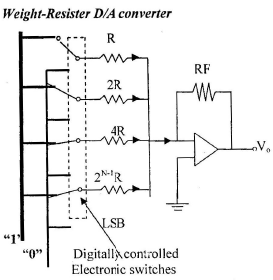Resister for MSB = R Resister for LSB = 2N–1.R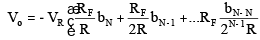Example : 4 bit D/A, V (1) = 1V, RF = 8R. Obtain analog output for 0101?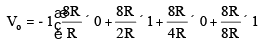D/A Performance Characteristics 1. FSV (full scale voltage): It represents maximum output voltage for the DA converter and is obtained when all bits of digital input are 1.
The output for any bit is e0 = VR/2n
where n = no. of bits.
FSV = VR [1 - (1/2n)]

2. Resolution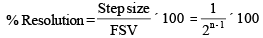3. Ccuracy: It is the error between the actual output of DA converter to the expected or theoretical output and expressed as percentage of FSV.
If the converter has an accuracy of ± 0.1% for FSV of 10 V, the minimum error for any output voltage is [(10/100) × 0.1] = 10 mV Ideally the accuracy should be = ± [1/2]1 LSB.

4. Monotonicity: It means that the output increase for an increase of input or decreases for decrease of input. The output should not decrease for increase of input. 5. Settling Time: It is the time taken by DA converter to settle within + [1/2] LSB of its final value.

Major component of the binary weighted resistance DAC:
a) a weighted resistor network, R to 2n-1 R
b) n switches, one for each bit applied bit input.
c) A reference voltage Vref
d) A summing element Defects:

The chief detect of weighted binary DAC is that the resistor values increases in multiples of 2.

If the value of MSB is 2 KW. then for a 10 bit, the value for LSB is 29. 2K = 1.024 MW.
It is difficult to obtain high precision at very high values. It is also difficult to fabricate high value resistance by integrated circuit methods. This is over come by the ladder type DA converter.

R-2R ladder Network In this, only two values of resistors are used.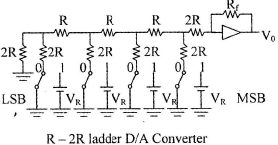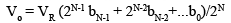The number of resistor required for an N-bit ladder D/A converter is 2N in the case of R-2R ladder D/A converter whereas it is only N is the case of weighted-resister D/A converter.

1. Parallel-comparator/flash/simultaneous A/D 

• Simplest in concept and fastest conversion time. 
• Disadvantages are rapid increase in the number of comparators with the number of bits, (2N-1) comparators are required for an N-bit converter.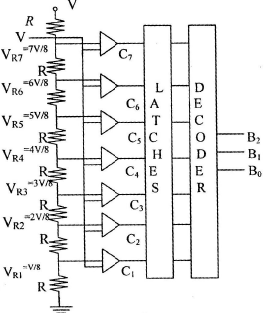2. Successive Approximation A/D converter 

• For N-bit converter, the number of clock pulses required would be N. 
• Slow than the parallel-comparator A/D Offset voltage = 1/2 LSB – 0.5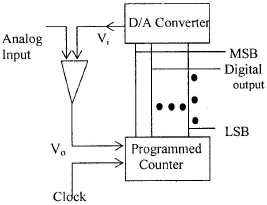3. Count ing A /D conver t er The maximum number of clock pulse required conversion is 2N for an N-bit A/D converter. This conveter is slower than the other two converters discussed earlier.

4. Dual-slope A/D converters Major component of a dual-slope A/D converter.
1. An integrator
2. A comparator
3. A binary counter
4. A switch driver.

1. Counter of Ramp type ADC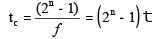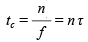3. Dual slope ADC Slowest conversion time 4. Flash ADC Fastest conversion time tc = (1/f) 

• Conversion time independent from no. of bit. 
• The number of Comparator = 2n–1 where f = clock frequency.

Aperture Time Aperture time is called the maximum allowable conversion time tc.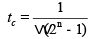where, w = angular frequency of signal applied
n = number of bit for ADC
ta = aperture time.

Offer running on EduRev: Apply code STAYHOME200 to get INR 200 off on our premium plan EduRev Infinity!

65 docs|37 tests

,

,

,

,

,

,

,

,

,

,

,

,

,

,

,

,

,

,

,

,

,

,

,

,

,

,

,

;